## Indicators

•Cited by SciELO
•Access statistics

•Cited by Google
•Similars in SciELO
•Similars in Google

## Print version ISSN 0123-3033

### Ing. compet. vol.16 no.1 Cali Jan./June 2014

Optimización del abastecimiento energético para usuarios no regulados en Colombia

Energy supply optimization for unregulated consumers

Diego F. Manotas-Duque
Escuela de Ingeniería Industrial, Grupo de Investigación en Logística y Producción UNIVALLE, Universidad del Valle, Cali, Colombia
E-mail: diego.manotas@correounivalle.edu.co

David A. Oliveros
Escuela de Ingeniería Industrial, Grupo de Investigación en Logística y Producción UNIVALLE, Universidad del Valle, Cali, Colombia
E-mail: daoliveros@hotmail.com

Héctor F. Taborda
Escuela de Ingeniería Industrial, Grupo de Investigación en Logística y Producción UNIVALLE, Universidad del Valle, Cali, Colombia
E-mail: hectabor@hotmail.com

Carlos J. Vidal-Holguín
Escuela de Ingeniería Industrial, Grupo de Investigación en Logística y Producción UNIVALLE, Universidad del Valle, Cali, Colombia
E-mail: carlos.vidal@correounivalle.edu.co

Carlos A. Lozano
Escuela de Ingeniería Eléctrica y Electrónica, Grupo de Investigación en Alta Tensión (GRALTA). Universidad del Valle, Cali, Colombia
E-mail: carlos.a.lozano@correounivalle.edu.co

Eje temático: Ingeniería industrial, Ingeniería eléctrica y electrónica / Industrial engineering, Electrical and electronic engineering
Recibido: 07 julio de 2012
Aceptado: 20 de septiembre de 2013

Resumen

Palabras Clave: abastecimiento energético, optimización del portafolio, usuarios no regulados, valor en riesgo condicional (CVaR).

Abstract

A supply electricity portfolio optimization model for unregulated consumers in the Colombian electricity market is proposed in this paper. The purpose is to choose between three supply alternatives available to the consumers: spot market purchase, purchase by bilateral contracts and self-generation, minimizing the total expected cost and the risk associated to these decisions. For this objective, a stochastic optimization model is used and the risk indicator is the conditional value at risk (CVaR). Finally, the model results are analyzed through the application of simulated prices based on real price observations from the database managed by XM - the Colombian Market Operator, and the best instance of risk aversion is selected.

Keywords: conditional value at risk, energy supply, portfolio optimization, unregulated consumers.

1. Introduction

The energy supply is a very important subject for companies because the cost of this service is usually a determinant factor of profitability margins. In the Colombian electricity market we can identify two different ways of energy supply: contracting with energy retailers and self-generation. For this work we have decided to include another energy supply option based on electricity spot market prices. This mechanism is possible, considering that some energy retailers transfer the risk associated with spot prices to the consumers through supply contracts based on this variable.

Supply based on electricity spot prices Some international electrical systems allow consumers to have direct access to spot markets to buy the energy supplied by the generators. In Colombia, this situation is not given explicitly because the regulatory framework prevents direct access of unregulated users to the spot market. Notwithstanding this observation, their access to the spot market is formalized through energy retailers through sale contracts based on spot prices plus a premium. The spot prices are conditioned by the interaction between energy demand and energy supply, but these variables are influenced by unpredictable elements that produce high electricity price volatility. Most of the energy generated in Colombia is provided by hydropower plants; therefore, the climate conditionsare a determinant factor of the energy offer (Álvarez & Tamayo, 2006). The electricity demand is influenced by the seasonality and it is different for each hour, day or month. These elements combined produce high electricity price volatility. In the energy supply based on spot markets, the consumer takes directly all price risks.

Contracting with energy retailers Unregulated users can establish contracts with retailers for medium and long terms (Álvarez & Tamayo, 2006). The purpose of these contracts is to include hedge instruments to reduce the electricity price risk. The contract prices for some periods show high values compared to spot prices, but contract prices are more stable. The hedge instrument used is the bilateral contract. This instrument can be considered as a forward contract.

Self-generation Some unregulated users may have power generation plants, which can be used to meet electricity consumption derived from productive activity. Usually, these plants use fossil fuels or gas steam cycles for generation. An important feature is that these generation facilities can be connected to the national interconnection system to sell the remaining energy produced. This condition is known as co-generation. The self-generation faces additional risks to the price of electricity. These risks are associated with the sale process of energy surplus to the system. Particularly, the cost of generation fuel used is a very critical factor. In this work, this factor was not considered as a source of risk.

An unregulated consumer in the Colombian electricity market may use the two supply options available according to regulation. However, considering the large number of variables and the high volatility level of electricity spot prices and the bilateral contract prices, it is necessary to formulate methods to solve this supply problem minimizing the total supply cost and the risk associated, seeking better cost management and generating value for the company. Summarizing, we considered in this model the following energy supply options: bilateral contracts with retailers based on spot prices, bilateral contracts with fixed prices, and self-generation.

There are several agents in the Colombian electricity market. The generators have the role to produce energy. The transmission company transports this energy from the power plants to the National Interconnected System (SIN) in high tension levels. The distributors carry this energy to final consumers in low tension levels. The retailers have the role of contracting with final consumers. The price formula for final users is presented in the Equation 1.

P(\$⁄kWh) = Generation cost + Transmission Cost + Distribution Cost + Retailer Cost + Other Charges (1)

As it can be seen in the formula, each agent influences a specific section of the final cost. The objective of this work is to optimize the generation and retailing costs, considering that the transmission, distribution and other charges are regulated.

According to Marnette, et al. (2009) the conditions of energy supply for capital intensive industries have changed since the electricity markets started their liberalization process. The opening to competition in some links of the electricity value chain presents big opportunities and challenges to seek energy efficiency and consequently minor cost of energy supply.

The model proposed in this paper corresponds to the typical portfolio optimization problem such as the model proposed by Conejo, et al. (2005). This model is focused in the determination of the optimal mix with the same electricity supply options considered in the Colombian electricity market. Carrion, et al. (2007) studied the same problem including stochastic elements and the conditional value at risk (CVaR) to have a hedge instrument for price volatility. Menniti, et al. (2008) analyzed a similar problem in the Italian electricity market. In that work, the authors propose the diversification of portfolios to cover the risk associated with the electricity price volatility. These authors did not consider financial instruments to cover the positions. Pinto, et al. (2007) studied the problem using real options analysis to define supply options in the Brazilian electricity market.

In general terms, the problem of energy supply for unregulated users has not been treated extensively in the literature. This is because each country has different mechanisms for the definition of these users, and the relatively low importance attributed to the energy supply problem in industrial enterprises. Xia, et al. (2008) wrote a seminal work in which they proposed a model of setting prices for large consumers in China. The proposed model is based on the determination of prices for bilateral contracts from optimizing hydropower resources available and seeking profit maximization of the power company. In this scheme, large consumers simply receive the set price of energy as an input from the expectation of the utility company. However, the model considers possible adjustments in the contracts signed from adjustments related to consumption levels of each user and price-demand elasticity.

Among the key elements to consider in energy supply models from the perspective of users are: self-generation option, consumers' risk aversion, and supply options in different markets. Zare, et al. (2010 a) analyzed different energy supply strategies using the theory of decision based on information gaps. The model considered includes power purchase options via bilateral contracts, spot market purchases and low self-generation option. The key variable in the analysis presented is the uncertainty about the price of electricity. Later, Zare et al. (2010 b) presented a stochastic optimization model for a supply problem considering multimarket supply and incorporating an assessment of the level of risk aversion of the end user.

The model developed incorporated consistent criteria for quantifying financial risk associated with various alternatives for energy supply, while taking into account the correlation between electricity prices in the different markets considered. Zare, et al. (2011) continued the previous work in the field of energy supply from the theory of information gap, considering two fundamental criteria. First, the robustness of the model based on the decision maker's learning curve with the problem of supply and second, the possibility of obtaining financial advantage of low prices in the spot market. In the analysis presented, the authors made some simplifications in modeling to facilitate the use of classical methods of financial risk assessment. It makes use of the variance-covariance matrix for matching the volatility in the prices of the various supply options considered, and they use a joint normal probability distribution to model the energy supply cost.

In this paper, the problem of supply in the Colombian market is analyzed using a portfolio optimization model that makes use of the three sources described above and that incorporates elements of risk aversion as those proposed by Conejo, et al. (2010). In the following sections we explain the optimization model and describe its implementation in the Colombian electricity market. Subsequently, we present the results of applying the model and conclusions.

2. Methodology

The methodology used in this article is based on the model proposed by Conejo, et al. (2010). These authors propose a model for minimizing electricity supply costs considering three alternatives: buy based on spot prices, buy based on purchasing contracts, and self-generation. Although the Colombian regulation prevents direct purchase on the spot market, the model is adaptive to the extent that it is assumed that the option to buy power from marketers using indexed supply contracts at spot prices, is comparable to a direct intervention in the market. This assuming the marketing premium over the spot price is negligible and does not exhibit additional volatility associated to these prices. In this paper we propose an electricity supply portfolio optimization model for an unregulated consumer in the Colombian electricity market.

2.1 Nomenclature and model variables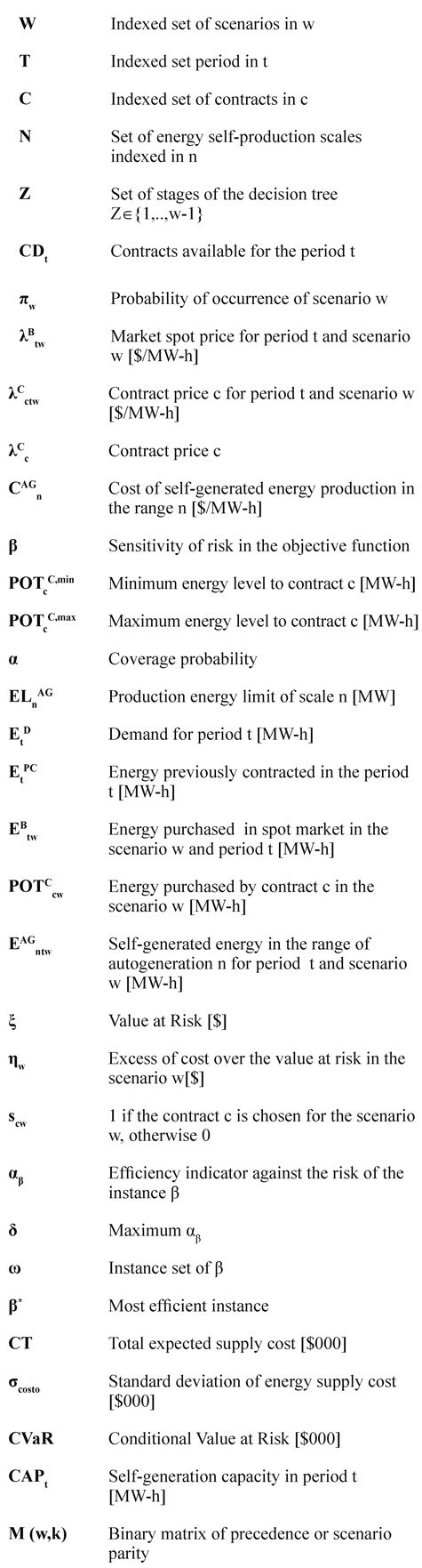2.2 Optimization model

The methodology is constructed based on a model of multi-stage stochastic optimization to determine the optimal portfolio of power supply that minimizes the total expected cost and risk associated value. We use a sensitivity parameter β to model different levels of risk aversion. The mathematical formulation of the proposed model is presented below: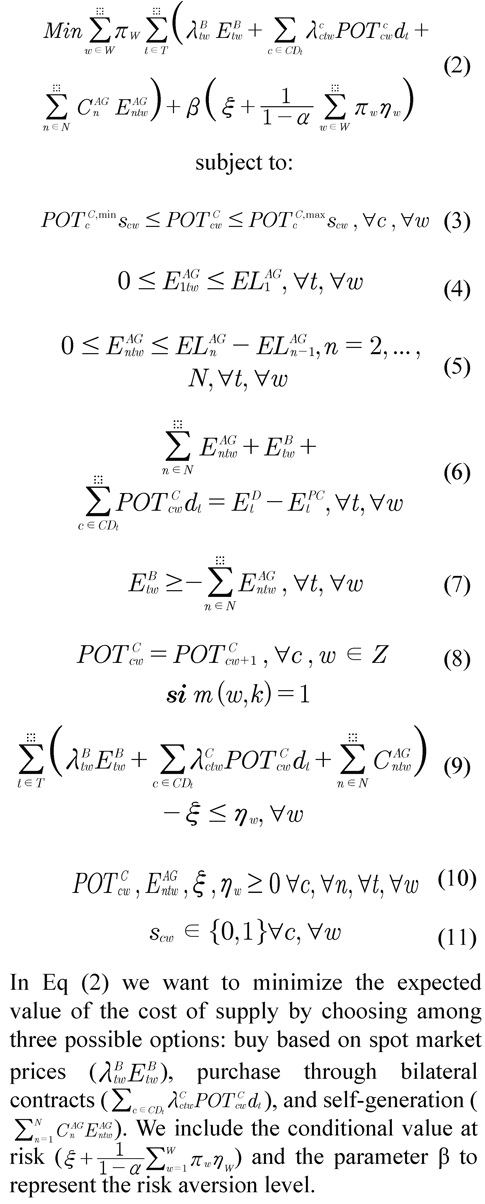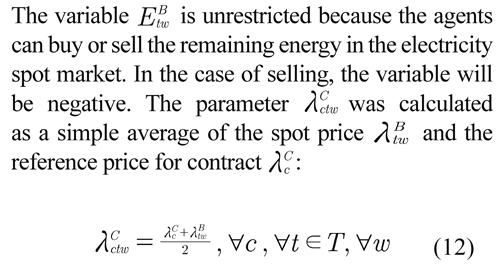Equation (3) establishes the power purchase contracts in accordance with the minimum and maximum limits for each option. In Equation (4) and Equation (5) we establish the limits of selfgeneration. For each limit or breaking point of the function, we can have a different production cost due to the effect of production scales (Figure 1).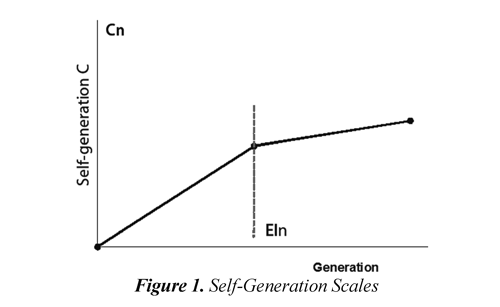Equation (6) establishes the balance between energy supply options and demand. The equation (7) restricts the possibility that the energy purchased through contracts can be sold by the user in the electricity spot market. Only self-generated energy can be used for this purpose.

The Equation (8) is proposed to constrain the decision variables associated to a node take identical values in the different scenarios that have the node as origin. This type of restriction is typical of stochastic programming problems. For this purpose a binary matrix M(w,k) is provided: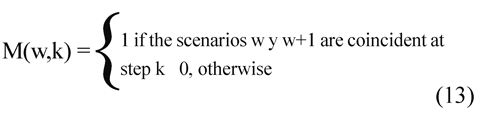Equation (9) provides the constraint associated to the calculation of conditional value at risk. Equation (10) shows the nature of the decision variables in the model and Equation (11) expresses the binary variable indicating whether a contract c is chosen on stage w. If this happens the variable takes the value of 1, otherwise 0.

2.3. Implementation of the model in the Colombian electricity market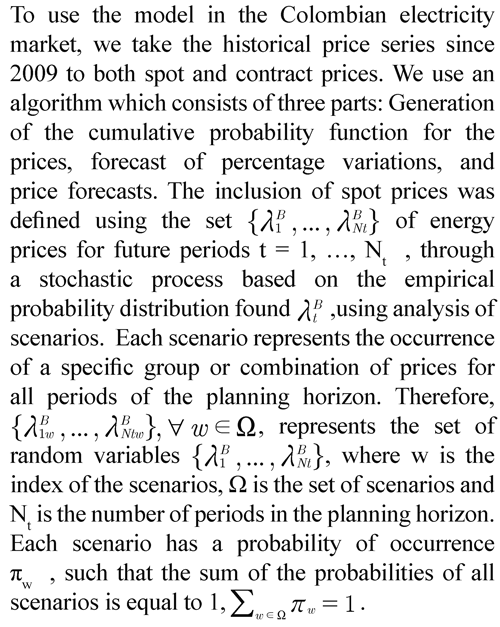With the data provided by the pricing algorithm, 64 scenarios with the same occurrence probability were built for a planning horizon of six months. The representation of market spot prices was performed using a decision tree, where each node is the starting point of two branches, each equivalent to a likely price for the period analyzed. The different price scenarios for all periods are obtained through the branches from the origin. The quantity of scenarios that must be considered in this diagram is a function of the number of periods of the planning horizon of the energy supply problem. If we consider six periods to analyze, the number of scenarios will be 26 = 64. In this model, the user has 8 bilateral contracts that can be considered: 2 of them available at the beginning of the horizon to be used in all periods and the remaining 6 contracts for use in each of the periods. The data for available periods, power limits and reference prices for each contract are specified in Table 1a. The value of parameter α (confidence level) used in the model is 0,95. The data of energy demand, capacity and unit cost of self-generation were established for a medium producer. This information is shown in Table 1b.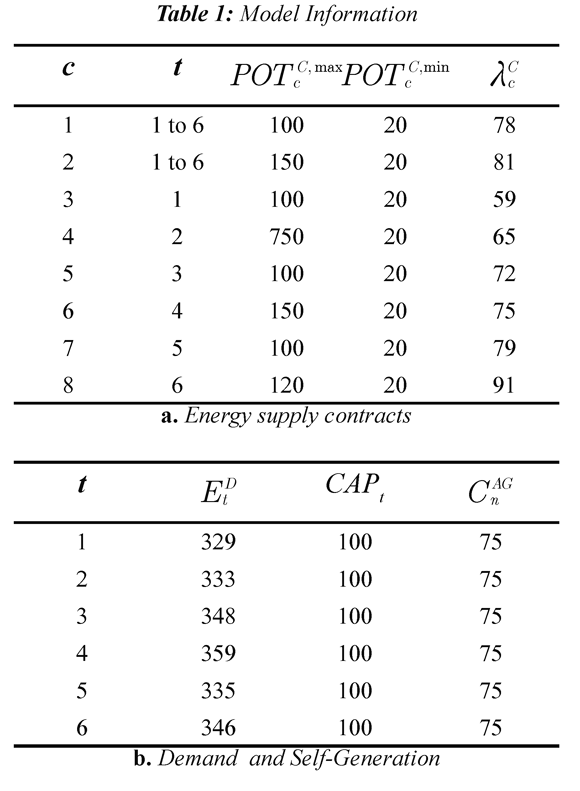According to the reference model used by Conejo et. al. (2010), the discretization of price generation process for each of the variables considered in the supply model facilitates the solution of the optimization problem but limits the reliability of the forecasting process. This deficiency is precisely one of the opportunities for improvement that result from this work, considering that the forecast is based on constant volatility models and it is clearly recognized that electricity prices exhibit characteristics of heteroskedasticity.

3. Results and discussion

The model was implemented in AMPL. The data shown in the previous section were included in the model. Additionally, we assess multiple instances of the model for different values of β. The results are presented below.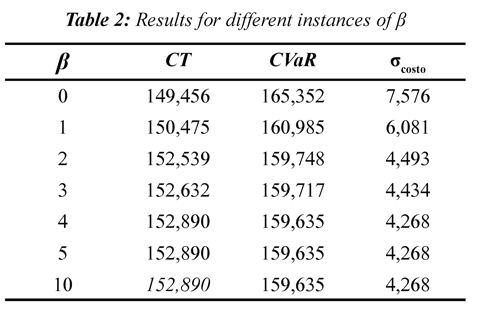We can observe in the results illustrated in Table 2, that as risk aversion increases (higher values of the parameter ß), the cost of energy supply increases, establishing a positive relationship between these two elements. Contrary to the cost of supply, indicators associated with risk, such as CVaR and s_costo decline as the level of risk aversion is higher. This occurs because supply decisions that are suggested with higher levels of risk aversion, involve greater participation of bilateral contracts and self-generation, reducing the volatility of the spot market prices. This behavior can be observed on Figure 2, showing the composition of the portfolio of supply for different instances of the parameter ß. In Figure 2 we can observe that at lower levels of risk aversion in the portfolio, the share of purchases in the electricity spot market increases.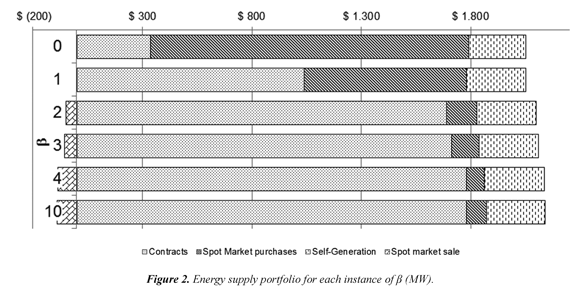On the other hand, for higher levels of risk aversion, energy purchases through bilateral contracts increase. Although direct supply through contracts has higher costs, it is justified when you consider that the volatility is lower, therefore bilateral contracts become effective hedging instruments for the user. The efficient frontier curves for this case show the inverse relation between total cost and the risk factors considered. (Figures 3 and 4).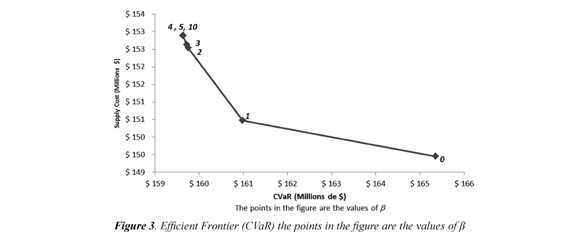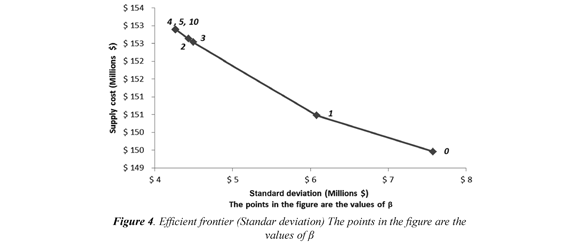We can see from the efficient frontier curves, that risk aversion levels that require relatively low additional costs may reduce the risk involved. For example, for values of β≥4 the supply cost remains constant showing an increase of 2.3% compared to the instance without risk aversion (β = 0).

For β = 4 the reduction of CVaR and the standard deviation is 3,5% and 43,5% respectively. We can observe, that when the value of β is equal to 1, we obtain a particularly relevant scenario, as it is possible to obtain a reduction of 20% in standard deviation compared to an increase of 0,7% on the amount of expected cost of supply by reference to the level without risk aversion. These results show the importance of this model for unregulated users, because we can establish the relationship between multiple variables in decisions about energy supply suggesting a portfolio that incorporates financial hedges against volatility of spot energy prices.

Up until the previous section we have discussed the results of the model and their relationship to risk aversion at different optimal portfolio decisions. To establish the best decision, there is a heuristic method that determines which is the best instance of β. The indicator used for the heuristic is defined as:The algorithm proposed for this heuristic method includes the following steps:

• We calculate the indicator αβ for each instance of β.
• Set ω = ᴓ}
• We calculate δ = argmaxβ}
• For each instance we assess ω←ω+{β|αβ= δ} β*
• We select the optimal instance = β|argmin{CTβϵω}

The indicator αβ establishes the relation between the total supply cost and the associated risk. When we analyze the indicator for a risk aversion level β = 0, αβ takes the minimum value because the CVaR, which operates as the denominator in the formula, corresponds to the largest possible amount of all scenarios. As higher risk aversion levels are considered (incremental values of the parameter β), the indicator αβ increases because the expected cost of supply (CTβ) becomes larger and thus CVaR decreases, which implies that scenarios that make the supply cost higher are being considered, which reduces the risk of experiencing higher costs due to the variability of spot energy prices. This decrease in the difference between the two factors makes it desirable to have a maximum αβ indicator, turning αβ into the ultimate criterion of choice when several β values agree on the indicator, the lower supply cost (CTβ).

It is important to reiterate that the decision stage should be guided not only by the single criterion of optimal total cost of supply, but the level of risk (CVaR) associated with each instance should also be considered and its minimization should be sought. Applying this heuristic to the results above, the optimal instance is β=3 (Table 3). This instance shows the maximum indicator αβ = 0,956. This is the most efficient instance in terms of cost and risk associated and it has the minimum energy supply cost. We can observe that the relation between cost and risk stabilizes from β = 3. If we consider the instance β = 4, the energy supply cost increases up to 152,89 millions, which would not be suitable considering that this instance maintains the same level of relative risk of the instance β = 3. There are scenarios with lower supply cost, β ≤ 2, however; having lower αβ indicators, these instances are not considered to have an efficient relationship between the power purchase cost and its associated risk.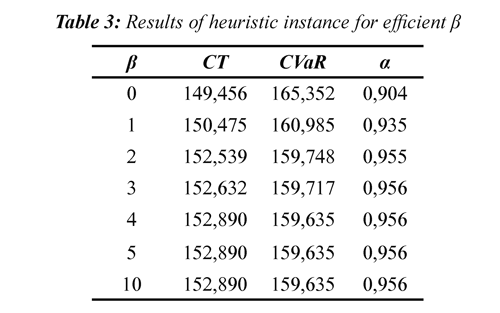The complete analysis of the case study determines that the best solution has an energy supply cost of \$152,63 millions with a CVaR equal to \$159,72 millions. This corresponds to an increase of \$3,18 million (2,13%) in the sourcing cost and a risk reduction, measured by CVaR, of \$5,64 million (3,41%), compared with the instance considered a nonexistent level of risk aversion. The overall portfolio of this solution is shown in Figure 5.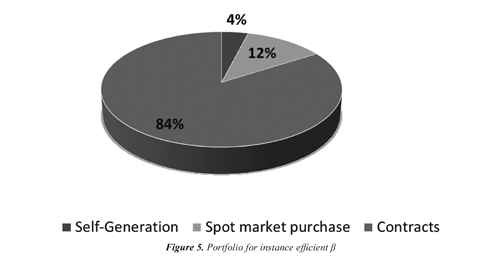4. Conclusions

The use of stochastic models to represent the energy supply problem for unregulated users allows us consider the behavior of very important variables in the analysis process, such as electricity spot prices, seeking more simple representations in deterministic contexts. The model applied to forecast electricity spot prices is a key element to obtain more reliable results in the supply mathematical model. Although there are advanced forecasting models that anticipate future behavior of electricity prices with minor deviations compared with the actual figures, this variable has characteristics of instability that makes it difficult to predict desirable levels with certainty.

The purchase of energy through bilateral contracts is usually more expensive but at the same time it reduces the transaction risk. In this context, the self-generation is another kind of financial hedge to face the volatility associated with electricity spot prices.

The biggest cost associated with those instances in which risk aversion levels are higher (higher β parameters), is because, in advance at the beginning of the planning horizon, it can choose by power purchase contracts that apply even in times when spot market prices may be lower than the reference prices of bilateral contracts.

The level of risk aversion that could be considered in the power purchase model by large consumers presents maximum limits, from which, the model does not produce changes in the target values and results obtained. To allow the inclusion of parameter values more adjusted to reality, it is very important that the stochastic model updates are made periodically according to the forecasted values with the objective to represent the dynamic of the problem under study.

The model can be adapted to other market situations with the purpose to include particular circumstances faced by users. For example, electricity spot markets with different structures, other types of energy supply contracts, different regulatory regimes, constraints of maximum limits of participation of power generation; and other price process. This point could be developed through the inclusion of supply contract price m Cctw in the price formation process.

5. References

Álvarez, S., & Tamayo, M. (2006). Descripción del Funcionamiento del Sector Eléctrico Colombiano. Ecos de Economía , 22, 7-44.         [ Links ]

Carrion, M., Philpott, A., Conejo, A., & Arroyo, J. (2007). A Stochastic Programming Approach to Electric Energy Procurement for Large Consumers. Power Systems, IEEE Transactions , 22 (2), 744-754.         [ Links ]

Conejo, A. J., Carrión, M., & Morales, J. M. (2010). Energy Procurement by Consumers. In, F. S. Hillier, & C. C. Price (Eds.) Decision Making Under Uncertainty in Electricity Markets (pp.323-355) New York: Springer.         [ Links ]

Conejo, A. J., Fernandez-Gonzalez, J. J., & Alguacil, N. (2005). Energy procurement for large consumers in electricity markets. Generation, Transmission and Distribution, IEEE Proceedings , 152 (3), 357-364.         [ Links ]

Marnette, W., Bausch, A., & Schwenker, B. (2009). The Energy Retail Market from a Customer Perspective. A. Bausch & B. Schwenker. Handbook Utility Management (pp. 591-604). Heildelberg, Germany: Springer Berlin-Heidelberg.         [ Links ]

Menniti, D., Musmanno, R., Scordino, N., & Sorrentino, N. (2008). Portfolio selection problem of a big consumer in the Italian electricity market. In IEEE. 3rd International Conference on Deregulation and Restructuring and Power Technologies (pp. 316-322). Nanjuing, China: IEEE.         [ Links ]

Pinto, L., Dias, B., Szczupak, J., Maia, R., & Tsunechiro, L. (2007). A novel risk management model based on the real options concept. In Proceedings at the IEEE POWERTECH (pp. 2144-2149). Lausanne, Switzerland.         [ Links ]

Xia, L., Junyong, L., Liang, D., & Zhang, L. (2008). Analysis on large consumer price models in electricity market. In IEEE. 3rd International Conference on Deregulation and Restructuring and Power Technologies (pp. 426-430). Nanjuing, China: IEEE.         [ Links ]

Zare, K., Conejo, A., Carrión, M., & Moghaddam, M. P. (2010) Multi-market energy procurement for a large consumer using a risk-aversion procedure. Electric Power Systems Research , 80 (1), 63-70.         [ Links ]

Zare, K., Moghaddam, M. P., & Sheikh El Eslami, M. K. (2010) Electricity procurement for large consumers based on Information Gap Decision Theory. Energy Policy , 38 (1), 234-242.         [ Links ]

Zare, K., Moghaddam, M. P., & Sheikh-El-Eslami, M. K. (2011) Risk-Based Electricity Procurement for Large Consumers. Power Systems, IEEE Transactions , 26 (4), 1826-1835.         [ Links ]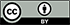Revista Ingeniería y Competitividad por Universidad del Valle se encuentra bajo una licencia Creative Commons Reconocimiento - Debe reconocer adecuadamente la autoría, proporcionar un enlace a la licencia e indicar si se han realizado cambios. Puede hacerlo de cualquier manera razonable, pero no de una manera que sugiera que tiene el apoyo del licenciador o lo recibe por el uso que hace.